# Quadratics Parts of a Parabola and Vertex Form

• Slides: 30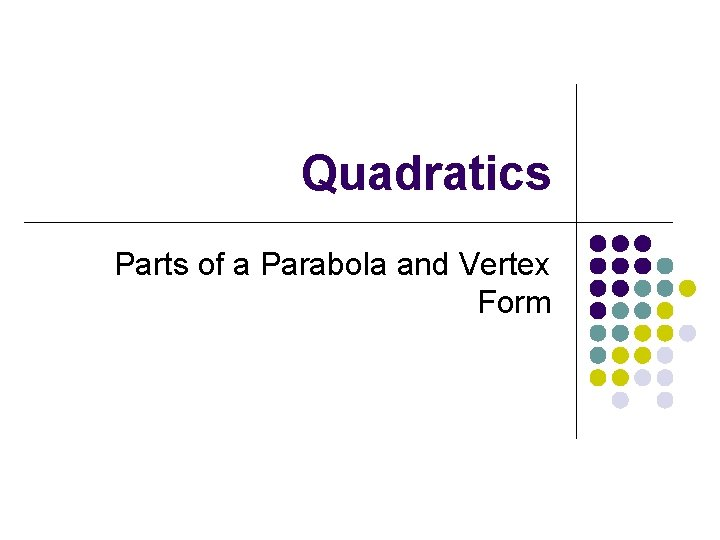Quadratics Parts of a Parabola and Vertex Form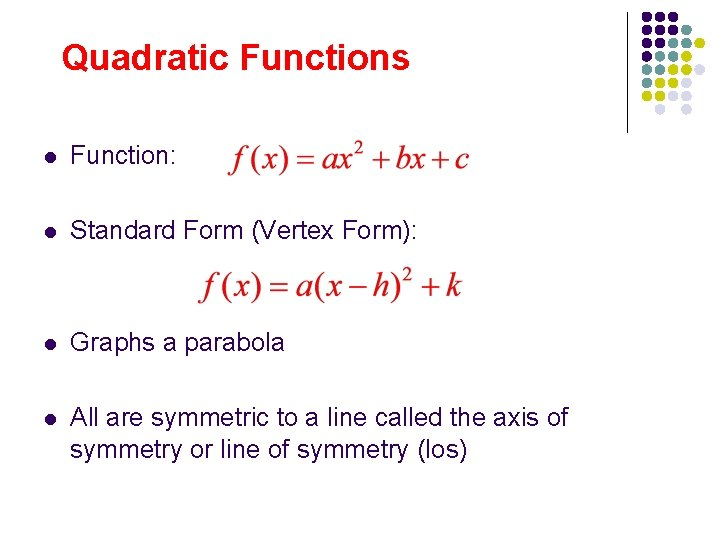Quadratic Functions l Function: l Standard Form (Vertex Form): l Graphs a parabola l All are symmetric to a line called the axis of symmetry or line of symmetry (los)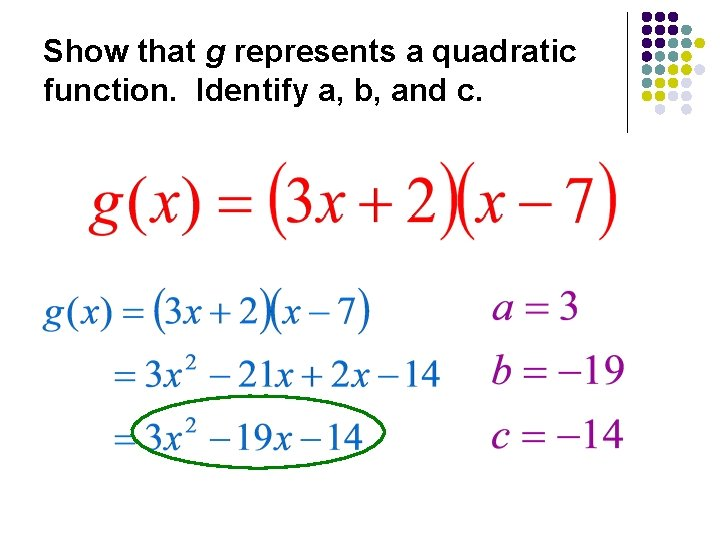Show that g represents a quadratic function. Identify a, b, and c.Show that g represents a quadratic function. Identify a, b, and c.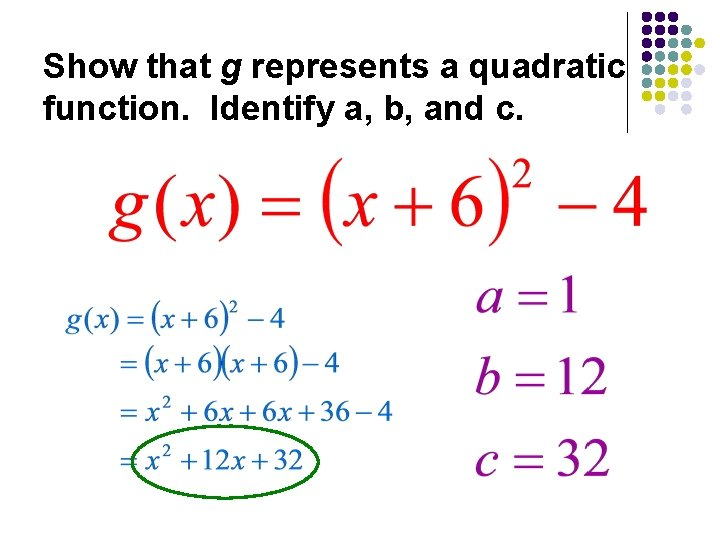Show that g represents a quadratic function. Identify a, b, and c.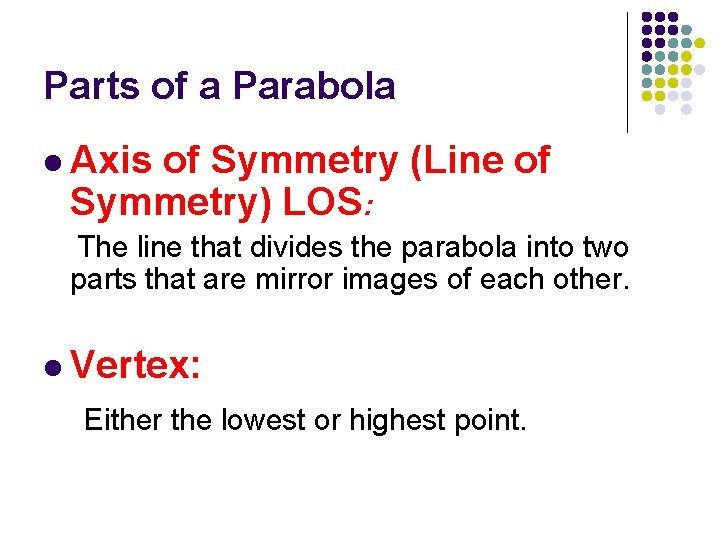Parts of a Parabola l Axis of Symmetry (Line of Symmetry) LOS: The line that divides the parabola into two parts that are mirror images of each other. l Vertex: Either the lowest or highest point.Let’s look at a transformation What is the vertex, max or min, and los?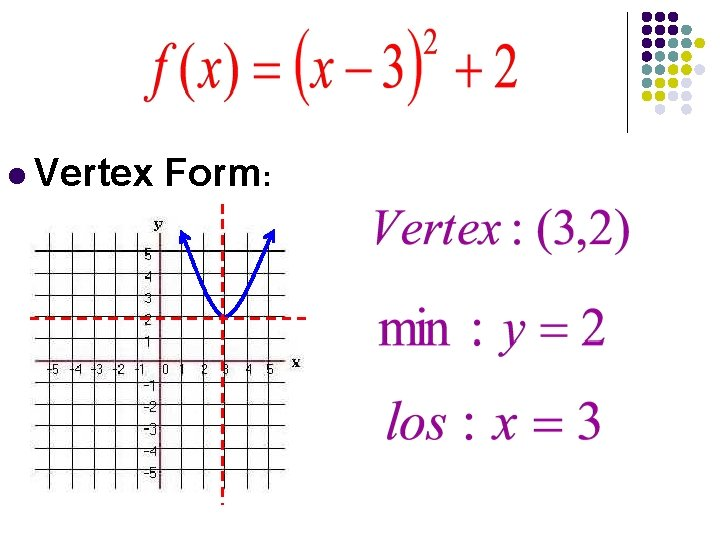l Vertex Form: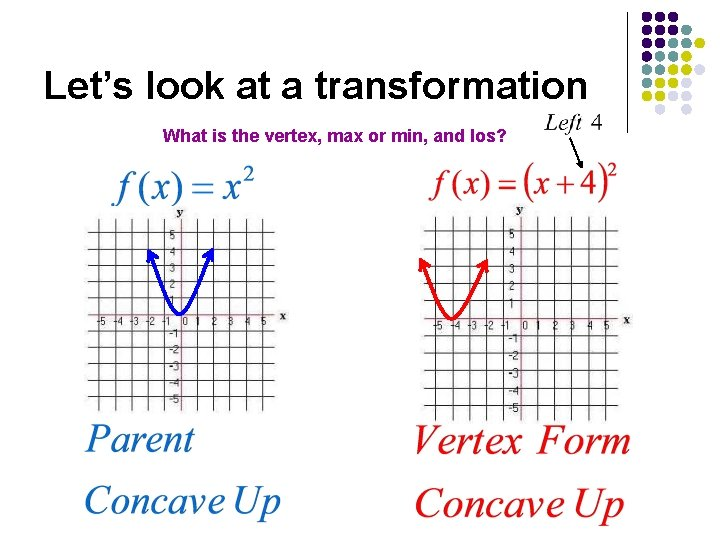Let’s look at a transformation What is the vertex, max or min, and los?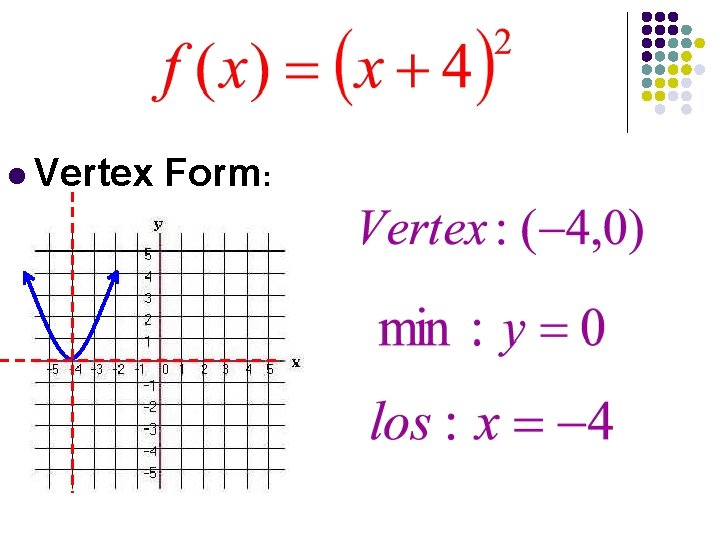l Vertex Form:Let’s look at a transformation What is the vertex, max or min, and los?l Vertex Form:Finding the Vertex and los on the GDC l l l Put equation into y 1. Press Zoom 6 – fix if necessary by changing the window. Press 2 nd Trace (Calc). Press max/min. Left of it; Then right of it; Then ENTER.Let’s Try It…. l Find the vertex, min, and los.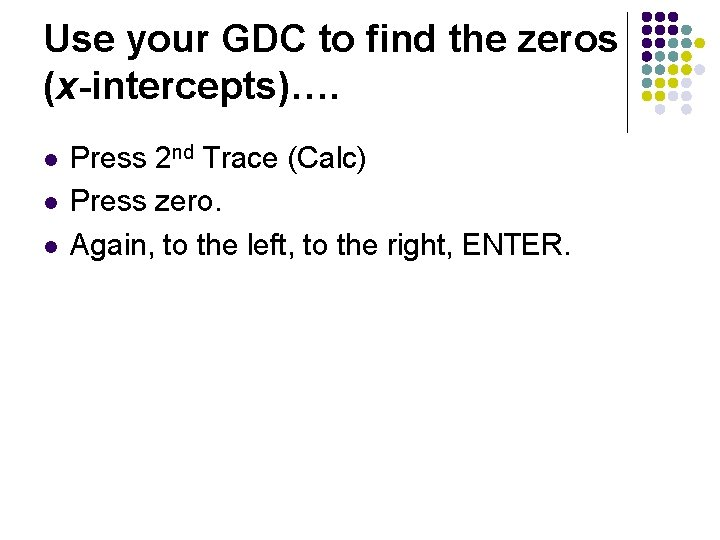Use your GDC to find the zeros (x-intercepts)…. l l l Press 2 nd Trace (Calc) Press zero. Again, to the left, to the right, ENTER.Find the zeros for the last example.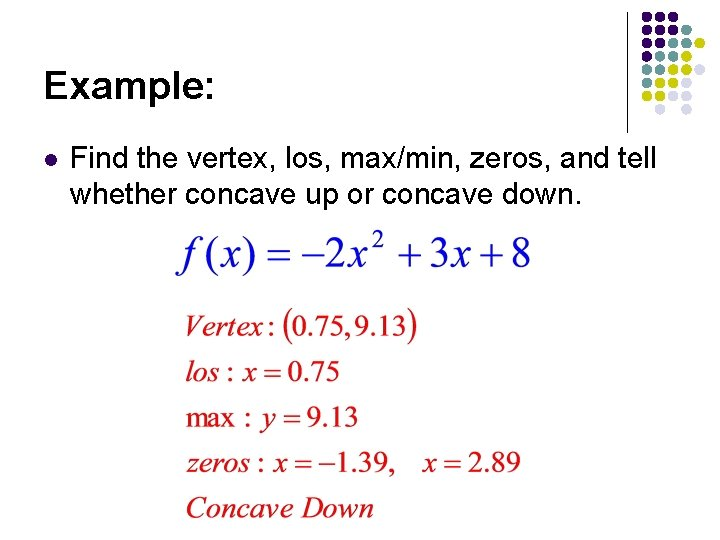Example: l Find the vertex, los, max/min, zeros, and tell whether concave up or concave down.Example: l Find the vertex, los, max/min, zeros, and tell whether concave up or concave down. These are the solutions. What are the x-intercepts?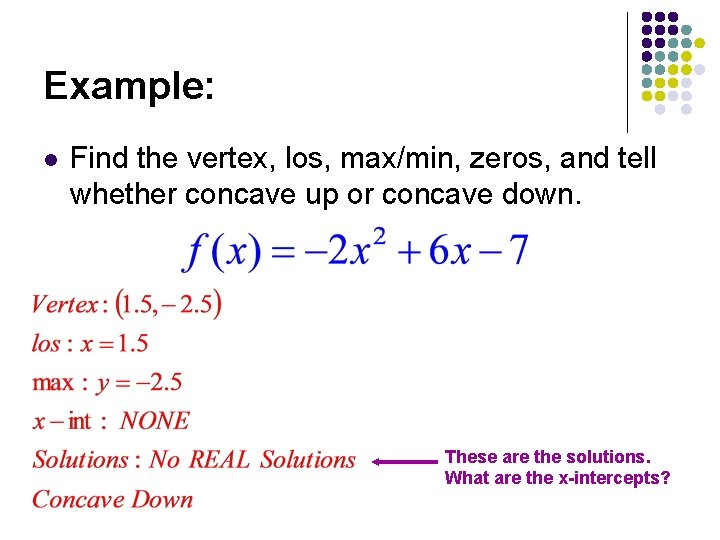Example: l Find the vertex, los, max/min, zeros, and tell whether concave up or concave down. These are the solutions. What are the x-intercepts?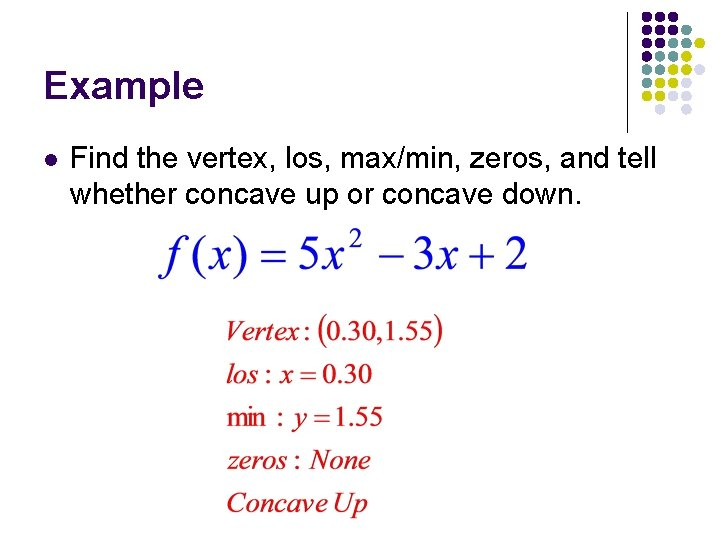Example l Find the vertex, los, max/min, zeros, and tell whether concave up or concave down.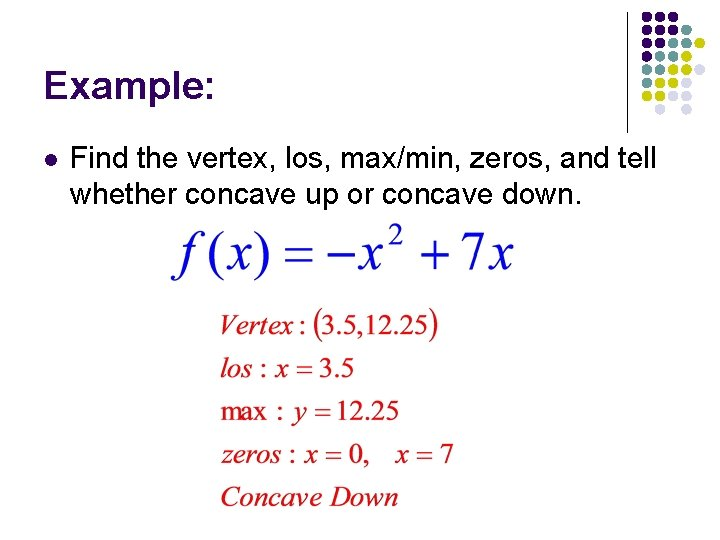Example: l Find the vertex, los, max/min, zeros, and tell whether concave up or concave down.Example: l Find the vertex, los, max/min, zeros, and tell whether concave up or concave down.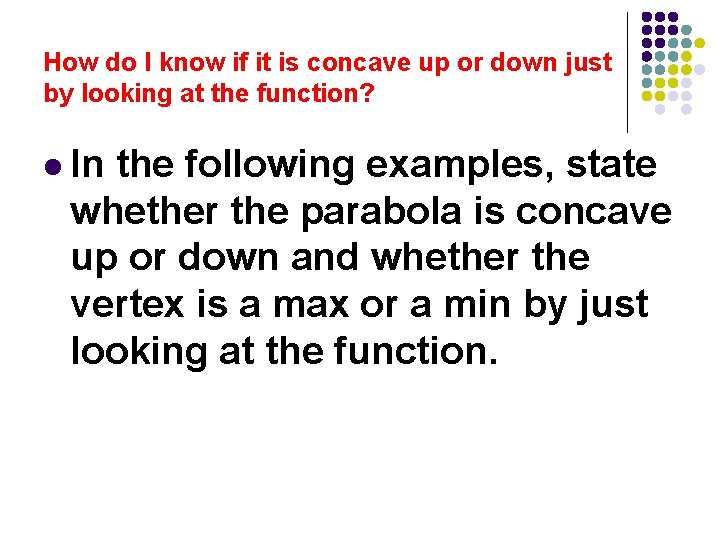How do I know if it is concave up or down just by looking at the function? l In the following examples, state whether the parabola is concave up or down and whether the vertex is a max or a min by just looking at the function.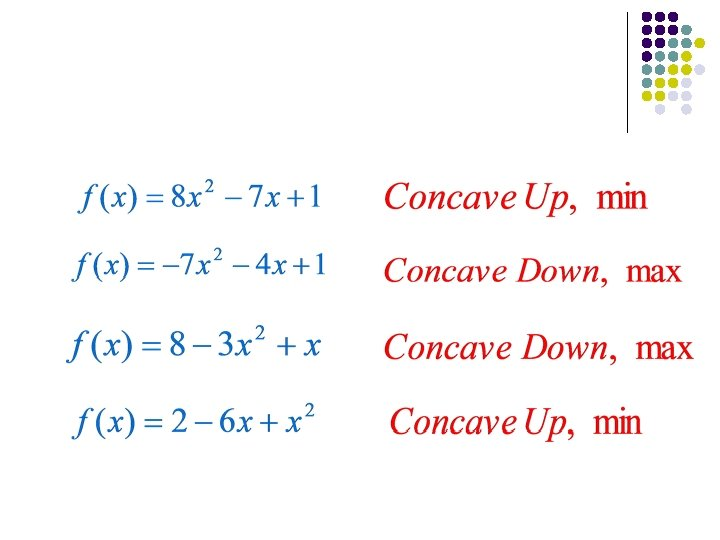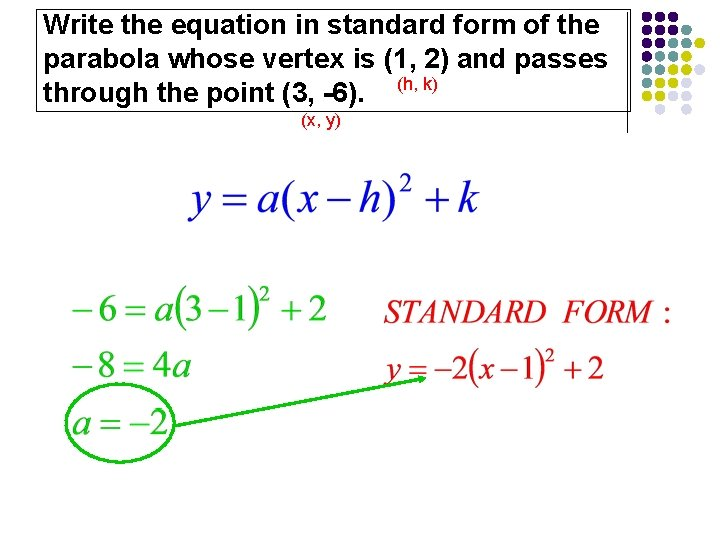Write the equation in standard form of the parabola whose vertex is (1, 2) and passes through the point (3, -6). (h, k) (x, y)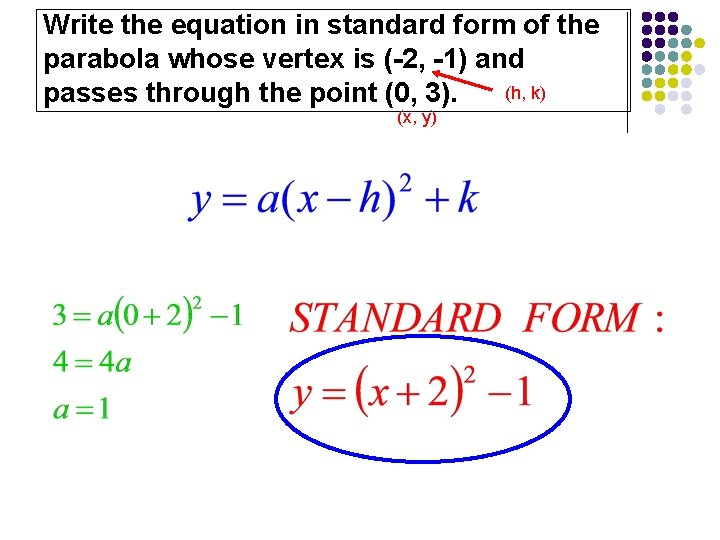Write the equation in standard form of the parabola whose vertex is (-2, -1) and (h, k) passes through the point (0, 3). (x, y)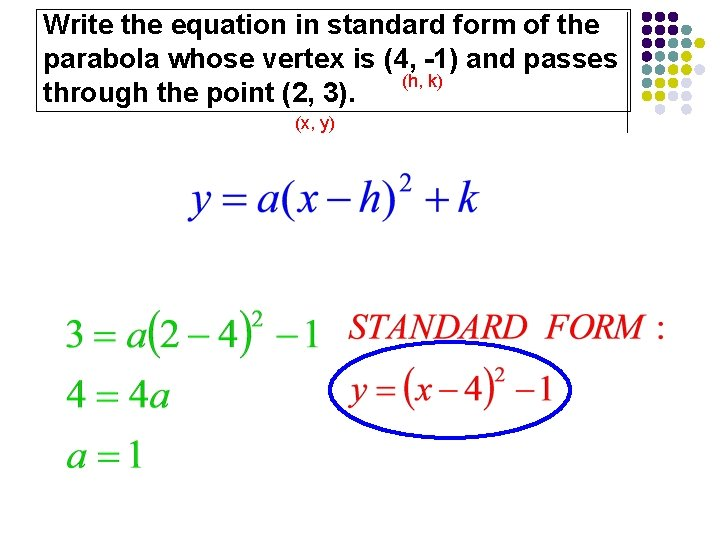Write the equation in standard form of the parabola whose vertex is (4, -1) and passes (h, k) through the point (2, 3). (x, y)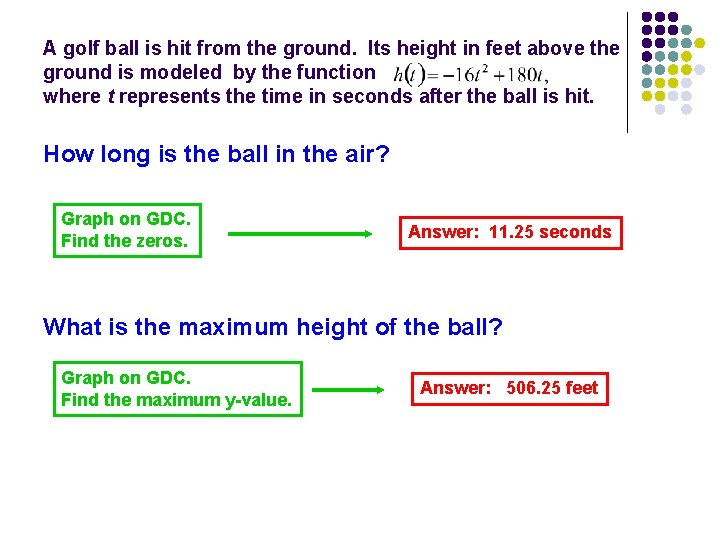A golf ball is hit from the ground. Its height in feet above the ground is modeled by the function where t represents the time in seconds after the ball is hit. How long is the ball in the air? Graph on GDC. Find the zeros. Answer: 11. 25 seconds What is the maximum height of the ball? Graph on GDC. Find the maximum y-value. Answer: 506. 25 feetl A. What is the maximum height of the ball? Graph and find the maximum y-value. Answer: 21 feet B. At what time does the ball reach its maximum height? Set equation = to 21 and find the zeros. Answer: 1 second C. At what time(s) is the ball 16 feet high in the air? Set equation = to 16 and find the zeros. Answer: 1. 56 seconds and 0. 44 seconds.§ . A. What ticket price gives the maximum profit? Graph and find the maximum x-value. Hint: Press Zoom 0 and change x-max to 50 and y max to 8000. Answer: \$25 B. What is the maximum profit? Graph and find the maximum y-value. Hint: Press Zoom 0 and change x-max to 50 and y max to 8000. Answer: \$6, 000 C. What ticket price would generate a profit of \$5424? Set equation = to 5424 and find the zeros. Hint: Press zoom 0. Answer: \$19 or \$31